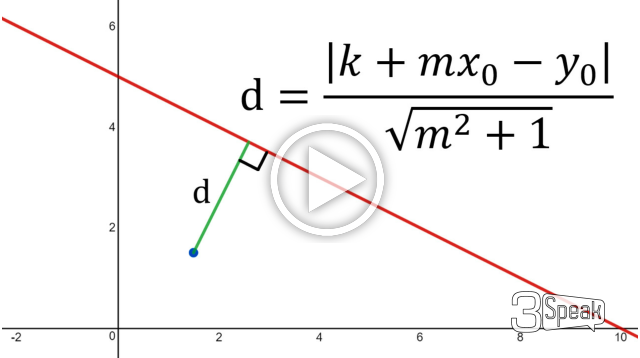# Point-To-Line Distance Formula: Alternative Formula

in Threespeak5 months ago (edited)In this video I once again tackle the formula for the distance from a point to a line but this time look at an alternative method and formula. Instead of using the general formula for a line, I use the more common formula, that is y = mx + k; where m is the slope and k is the y-intercept. Using this formula and the fact that the shortest distance from a point to a line is itself a perpendicular line we can then equate the two lines to solve for the intersecting point. Since perpendicular lines are negative reciprocals of each other, we can then solve for the intersecting point in terms of m, k, and the coordinates of the main point we are looking at. Combined with the Pythagorean Theorem to obtain the distance formula, we then need to undergo some algebra to finally get the distance formula. This formula is actually the same as the one solved in my earlier Algebraic and Geometric Proofs and we can show this by re-arranging the general ax + by + c =0 formula into y = mx + k form. Note also that just as in my earlier proofs, the proof itself if valid only for slanted lines but the final result is nonetheless applicable for horizontal and vertical lines. This is a very good video showing the many different methods of solving the same problem, so make sure to watch it!

Download the notes in my video: https://1drv.ms/b/s!As32ynv0LoaIhv99YxZ9t2THorI4hQ

View video notes on the Hive blockchain: https://peakd.com/mathematics/@mes/video-notes-point-to-line-distance-formula-alternative-formula

Related Videos:

Point-To-Line Distance Formula: Geometric Proof #1: https://youtu.be/9ilcXZn9CfE
Point-To-Line Distance Formula: Algebraic Proof: https://youtu.be/tngPrM2d-OM
Simple Proof of the Pythagorean Theorem: http://youtu.be/yt-EJlbJQp8
Negative Reciprocals and Perpendicular Lines: http://youtu.be/Ue7FmrfmuX4 .

SUBSCRIBE via EMAIL: https://mes.fm/subscribe

DONATE! ʕ •ᴥ•ʔ https://mes.fm/donate

Like, Subscribe, Favorite, and Comment Below!

Follow us on:

MES Truth: https://mes.fm/truth
Official Website: https://MES.fm
Hive: https://peakd.com/@mes
Gab: https://gab.ai/matheasysolutions
Minds: https://minds.com/matheasysolutions
Twitter: https://twitter.com/MathEasySolns
Facebook: https://fb.com/MathEasySolutions
LinkedIn: https://mes.fm/linkedin
Pinterest: https://pinterest.com/MathEasySolns
Instagram: https://instagram.com/MathEasySolutions
Email me: contact@mes.fm

Free Calculators: https://mes.fm/calculators

BMI Calculator: https://bmicalculator.mes.fm
Grade Calculator: https://gradecalculator.mes.fm
Mortgage Calculator: https://mortgagecalculator.mes.fm
Percentage Calculator: https://percentagecalculator.mes.fm

Free Online Tools: https://mes.fm/tools

iPhone and Android Apps: https://mes.fm/mobile-apps

▶️ 3Speak